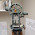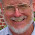## Thursday, October 6, 2011

### RPL Programming Tutorial - Part 2 - HP 49g+/50g: Local Variables

Welcome!

Welcome to Part 2 of the RPL Programming Tutorial for the HP 49g+ and 50g calculators. To recap, Part 1 talked about how to create, save, run, and (if need be) delete a program.

In Part 2, introduces the concept of the local variable. Simply put, a local variable is a variable that is used in a program and is then purged (deleted) at the end of the program. Local variables are not stored outside of the program, which saves memory.

A general structure of declaring local variables goes like this:

<< commands needed to set up the local variables, if any &rarr Local Variables
<< main program >> >>

=================================================================
Hint: There are times that you want to store information outside of a program. To do so, just store the data in a variable enclosed in single quotes followed by a STO command. These variables are called global variables.
=================================================================

Fibonacci Sequence

The well known Fibonacci Sequence is:
1, 1, 2, 3, 5, 8, 13, 21, 34,...

After the first two numbers, each succeed number is the sum of the last two numbers.

F_n = F_(n-1) + F_(n-2) where F_1 = 1 and F_2=1.

You can quickly find the mth term of the Fibonacci Sequence by using this closed formula:

f(n) = (ø^(n + 2) - (1 - ø)^(n + 2)) ÷ √5

Where:
ø = (1 + √5) ÷ 2, the Golden Ratio
n = m - 2

There are two local variables used in this program:
N = M - 2, the user supplies where M is the desired mth term
H = ø

The Program FIBN

Comments will be italicized, starting with an asterisk *. This program starts with M on Level 1 of the stack.

[RS] [ + ] (<< >>)
* Start of the program
2 [ - ]
* Subtract 2 from M to get N
1 [SPC] 5 [ √ ] [ + ]
2 [ ÷ ]

* Set up the Golden Ratio Constant, to be stored in H
[RS] [ 0 ] (&rarr)
* Prepare to name the local variables
[ALPHA] [EVAL] (N) [SPC]
[ALPHA] [MODE] (H)
[RS] [ + ] (<< >>)

* Start the main program
[ALPHA] [MODE] (H) [SPC]
* Leave spaces in between H and N
[ALPHA] [EVAL] (N) [SPC]
2 [ + ] [y^x]

* H^(N + 2); [y^x] is shown as ^
1 [SPC] [ALPHA] [MODE] (H) [ - ]
[ALPHA] [EVAL] (N) [SPC] 2
[ + ] [y^x]

* (1 - H)^(N + 2)
[ - ]
* H^(N + 2) - (1 - H)^(N + 2)
5 [ √ ] [ ÷ ]
* ( H^(N + 2) - (1 - H)^(N + 2) ) ÷ √5
[EVAL]
[ENTER]
* To terminate program entry

[ ' ] [ALPHA] [ALPHA]
[F6] (F) [TOOL] (I) [F2] (B) [EVAL] (N)
[ENTER] [STO>]

Here is the completed program:

<< 2 - 1 5 √ + 2 / → N H
<< H N 2 + ^ 1 H - N 2 + ^ - 5 √ / EVAL >> >>

How to run FIBN:
1. Enter M on the Stack
2. Run FIBN

Results:
FIBN(1) = 1
FIBN(2) = 1
FIBN(3) = 2
FIBN(4) = 3
FIBN(5) = 5
etc...

That wraps up Part 2. In Part 3, the IF-THEN-END and IF-THEN-ELSE-END program structures are introduced. Until then, Cheers! Eddie

Source Used: Math Formulas and Tables from Mobile Reference. SoundTells, LLC 2003-2010

This tutorial is created and is the property of Edward Shore. No mass reproduction without express permission of the author.

Edit: 10/20/11: correct an error in the formula in the text. The program is correct.

1.Great example!!! Thanks Eddie.

2.Hi Eddie
I love your tutorials. I used to be very familiar with HP41 programming, but the HP50g is so much different!
I want to write a simple program that takes a year (e.g. 2015) off Level 1, stores that as variable 'Y', divides it by 19, takes the fractional part, multiplies the FP by 19 to get the remainder as a real number, and store that as variable 'a'.
If I run <<19 / FP 19 * >>, no problem, and the number 1 is left on Level 1. The moment I try to create a variable, I get "STO Error: Bad Argument Type".
For example, << STO 'Y' 19 / FP 19 * >> and << 19 /FP 19 * STO 'a' >> bothe generate the error report, so obviously I'm doing something wrong with the STO command.
Where am I going wrong, please?

1.I think I've solved the problem myself. I omit the STO commands from the program, and the numbers are automatically stored in the named local variables which are created each time the program is run.
So, the program now reads: << 'Y' 19 / FP 19 * 'a' >>.

3.Me again - two years later.
4.5.